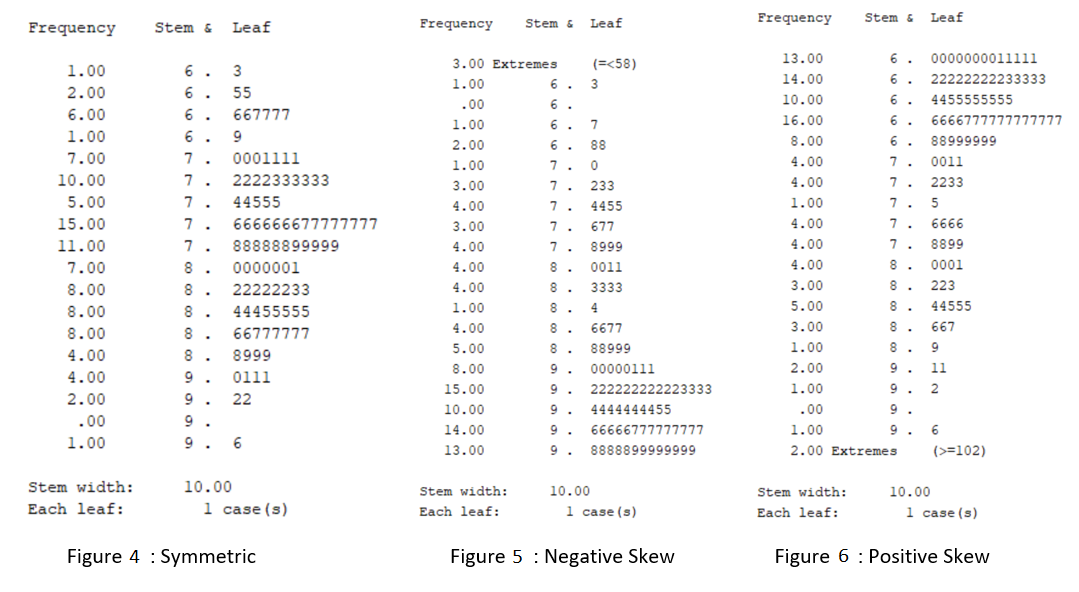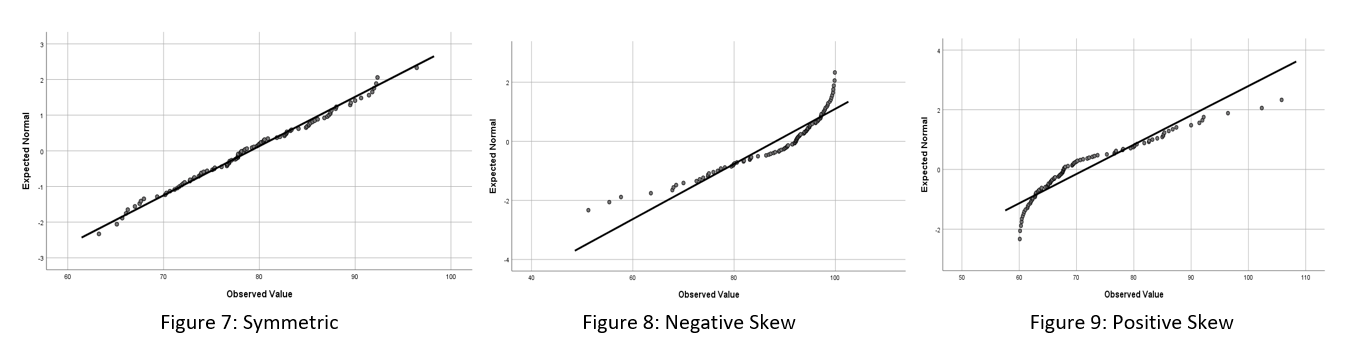# Introduction to statistics

Many of the statistics detailed in the Inferential statistics page of this module rely on the assumption that continuous data approximates a normal distribution. Hence knowing what the normal distribution is and how to test for it is very important, and is covered in this page first.

## Properties of the normal distribution

The normal distribution is a special kind of distribution that large amounts of naturally occurring continuous data (and hence also smaller samples of such data) often approximates. As a result, properties of the normal distribution are the underlying basis of calculations for many inferential statistical tests (called parametric tests). These key properties are as follows:

• the mean, median and mode are all equal; and
• fixed proportions of the data lie within certain standard deviations of the mean; 68% within one SD, 95% within two SDs and 99.7% within 3 SDs.

Hence the histogram for a normally distributed variable has a bell shape as shown below (note the percentages displayed here are given to two decimal places, while the percentages above are rounded values; also note that the $$\mu$$ and $$\sigma$$ symbols represent population mean and population standard deviation respectively):If you would like to practise interpreting a normally distributed data set, have a go at the following activity:

## Testing for normality

Since many inferential statistical tests rely on the assumption that a sample of continuous data approximates a normal distribution, it is important to be able to test for this. Unfortunately though there is not a single yes-or-no test for normality, and rather it requires assessing up to eight different factors in order to determine if the data approximates the normal distribution ‘closely enough’ (the data will never be perfectly normally distributed, and often a fair bit of deviation is acceptable). While some of these tests are more commonly used than others, it is a good idea to evaluate as many as possible, particularly when you are first getting started, as the more information you have means the more complete of a picture you will have of your data, and the more well-informed your conclusion will be.

The eight statistics and graphs you can interpret, in no particular order, are as follows:

• Mean, median and mode
For a perfect normal distribution these three values should all be the same, so checking whether they are similar is a good (and simple) way to start. Note however that even in normally distributed data the mode may sometimes be higher or lower, but this is less of a concern than any differences between the mean and median.

• Skewness
Skewness indicates the direction of any tail of the histogram. If the data is normally distributed the skewness should be close to $$0$$ ($$0$$ indicates a perfect normal distribution), but at least in the range of $$-1$$ to $$1$$ (negative values indicate negative skew with the tail to the left, i.e. skewed to the left; positive values indicate positive skew with the tail to the right, i.e. skewed to the right). A z-score can also be calculated for skewness by dividing the skewness by its standard error, and this should be within the range of $$-1.96$$ to $$1.96$$.

• Kurtosis
If the data is normally distributed the kurtosis should be close to $$0$$, but at least in the range of $$-1$$ to $$1$$ (positive kurtosis indicates a high peak around the mean and fatter tails; negative kurtosis indicates a lower peak around the mean and thinner tails). A z-score can also be calculated for kurtosis by dividing the kurtosis by its standard error, and this should be within the range of $$-1.96$$ to $$1.96$$.

• Normality test (i.e. Shapiro-Wilk)
This test is generally only used for sample sizes less than $$100$$ as it can be too sensitive for larger samples. If you do use it note that it tests the null hypothesis that the distribution approximates a normal distribution, so a significance ($$p$$) value greater than $$0.05$$ is typically required (more on hypothesis testing in the Inferential statistics page).

• Histogram
If the data is normally distributed the histogram should be approximately symmetric and centred around the mean (Figure 1). Alternatively if there is a long tail to the left only we say it is skewed to the left (negatively skewed) (Figure 2); or if there is a long tail to the right only we say it is skewed to the right (positively skewed) (Figure 3):• Stem and leaf plot
A stem and leaf plot displays the frequency of each value in the data set, organised into ‘stems’ and ‘leaves’. For example, Figure 4 below shows that there is one value of $$63$$, two values of $$65$$, six values of either $$66$$ or $$67$$, etc. While this plot is less frequently analysed, if you do choose to use it note that it can be interpreted in the same way as a histogram, only rotated on its side.• Normal Q-Q plot
If the data is normally distributed the points on a normal Q-Q plot will fall approximately on the straight diagonal line (Figure 7). Otherwise, the points will not lie on the straight diagonal line (Figures 8 and 9). Note that another version of this plot, the detrended Q-Q plot, is sometimes also analysed; in the detrended plot there should be roughly equal number of points above and below the line, with no obvious trend.• Boxplot

If the data is normally distributed the median should be positioned approximately in the centre of the box, both whiskers should have similar length and ideally there should be no outliers (Figure 10). Alternatively, the variable may be negatively skewed (Figure 11) or positively skewed (Figure 12):After analysing the data relating to these tests of normality, you should come to an overall conclusion based on what the majority of the tests indicates. For example, your conclusion might be that the data is approximately normally distributed, or it might be that it is positively or negatively skewed.

For a worked example of assessing normality, you make like to view the Introduction to SPSS module. You can also practise assessing whether or not data approximates a normal distribution by having a go at the following activity:

## Transforming variables

If tests for normality indicate that the variable is not normally distributed, you can try transforming the variable so that it conforms more to the normal distribution.

To transform a skewed continuous variable, you can apply:

• Natural logarithms (i.e. $$ln$$) - to correct a positively skewed continuous variable (most commonly used)
• Square root - to correct a positively skewed continuous variable
• Reciprocal - to correct a positively skewed continuous variable
• Squares - to correct a negatively skewed continuous variable

Once the data has been transformed, it should be tested again for normality. If the transformation has ‘worked’, any further inferential analysis should be conducted on the transformed data. If it hasn’t, you will need to use non-parametric tests instead.